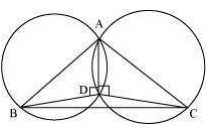# ABC and ADC are two right triangles with common hypotenuse AC. Prove that ∠ CAD = ∠CBD.Given:

ABC and ADC are two right-angle triangles with common hypotenuse AC.
To prove:

Solution:

The circle is drawn by taking AC as diameter passes through B and D.

We know that the angles which are in the same segment of the circle are equal.

∴ ∠ CAD = ∠ CBD